1. /
2. CBSE
3. /
4. Class 12
5. /
6. Mathematics
7. /
8. CBSE Question Paper 2014...

# CBSE Question Paper 2014 class 12 Mathematics

CBSE Question Paper 2014 class 12 Mathematics conducted by Central Board of Secondary Education, New Delhi in the month of March 2014. CBSE previous year question papers with solution are available in myCBSEguide mobile app and cbse guide website. The Best CBSE App for students and teachers is myCBSEguide which provides complete study material and practice papers to cbse schools in India and abroad.

CBSE Question Paper 2014 class 12 Mathematics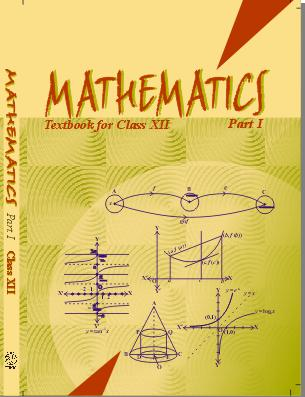## Class 12 Mathematics list of chapters

1. Relations and Functions
2. Inverse Trigonometric Functions
3. Matrices
4. Determinants
5. Continuity and Differentiability
6. Application of Derivatives
7. Integrals
8. Application of Integrals
9. Differential Equations
10. Vector Algebra
11. Three Dimensional Geometry
12. Linear Programming
13. Probability

## CBSE Question Paper 2014 class 12 Mathematics

### General Instruction:

• All question are compulsory.
• The question paper consists of 29 questions divided into three sections A, B and C.Section – A comprises of 10 questions of one mark each, Section -B comprises of 12 questions of four marks each and Section – C comprises of 7 questions of six marks each.
• All questions in Section – A are to be answered in one word, one sentence or as per the exact requirement of the question.
• There is no overall choice. However, internal choice has been provided in 4 questions of four marks each and 2 questions of six marks each. You have to attempt only one of the alternatives in all such questions.
• Use of calculators is not permitted. You may ask for logarithmic tables, if required.

### Section A

1. If A is a square matrix such that A2 = A, then write the value of 7A – (I + A)3, where I is an identity matrix.

2. If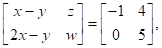find the value of x + y.

3. If tan-1 x + tan-1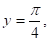xy < 1, then write the value of x + y + xy.

4. If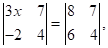find the value of x.

5. If f(x)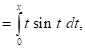then write the value of f’(x).

6. Find the value of ‘p’ for which the vectors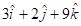and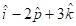are parallel.

7. If R = {(x, y) : x + 2y = 8} is a relation on N, write the range of R.

8. If the cartesian equations of a line are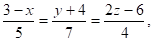write the vector equation for the line.

9. If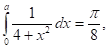find the value of a.

10. If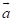and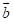are perpendicular vectors,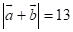and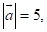find the value of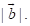### SECTION – B

11. Solve the differential equation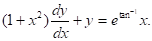12. Show that the four points A, B, C and D with position vectors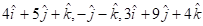and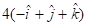respectively are coplanar.

### OR

The scalar product of the vector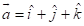with a unit vector along the sum of vectors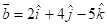and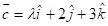is equal to one. Find the value of λλ and hence find the unit vector along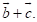13. Evaluate: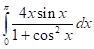### OR

Evaluate: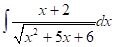14. Find the value(s) of x for which y = [x (x – 2)]2 is an increasing function.

### OR

Find the equations of the tangent and normal to the curve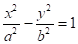at the point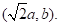15. If the function f : R → R be given by f(x) = x2 + 2 and g : R → R be given by g(x)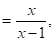x ≠ 1, find fog and gof and hence find fog (2) and gof (– 3).

These are questions only. To view and download complete question paper with solution install myCBSEguide App from google play store or login to our student dashboard.

## Mathematics Question Paper 2014 with solution

Download class 12 Mathematics question paper with solution from best CBSE App the myCBSEguide. CBSE class 12 Mathematics question paper 2018 in PDF format with solution will help you to understand the latest question paper pattern and marking scheme of the CBSE board examination. You will get to know the difficulty level of the question paper. CBSE question papers 2014 for class 12 Mathematics have 15 questions with solution.

## CBSE Question Paper 2014

CBSE question papers 2018, 2017, 2016, 2015, 2014, 2013, 2012, 2011, 2010, 209, 2008, 2007, 2006, 2005 and so on for all the subjects are available under this download link. Practicing real question paper certainly helps students to get confidence and improve performance in weak areas.

To download CBSE Question Paper 2014 class 12 Accountancy, Chemistry, Physics, History, Political Science, Economics, Geography, Computer Science, Home Science, Accountancy, Business Studies and Home Science; do check myCBSEguide app or website. myCBSEguide provides sample papers with solution, test papers for chapter-wise practice, NCERT solutions, NCERT Exemplar solutions, quick revision notes for ready reference, CBSE guess papers and CBSE important question papers. Sample Paper all are made available through the best app for CBSE students and myCBSEguide website.Test Generator

Create question paper PDF and online tests with your own name & logo in minutes.myCBSEguide

Question Bank, Mock Tests, Exam Papers, NCERT Solutions, Sample Papers, Notes